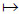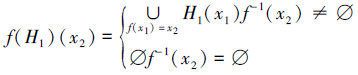﻿ 新型软域Download PDF文章快速检索 高级检索

1. 江南大学理学院, 江苏无锡 214122;
2. 江南大学智能系统与网络计算研究所, 江苏无锡 214122

New type of soft fields
ZHANG Longxiang1, LIAO Zuhua1,2, WANG Qi1, WANG Ruiyun1, LIU Weilong1
1. School of Science, Jiangnan University, Wuxi 214122, China;
2. Institute of Intelligence System & Network Computing, Jiangnan University, Wuxi 214122, China
Abstract: To develop a concept for a new type of soft field, we introduce a method that endows a parameter set with a field algebra structure that differs from that of traditional soft algebras. We then obtain the necessary and sufficient condition of a new type of soft field. By performing a soft-set intersection operation, we prove that the intersection operation of two new soft-field types still represents a new type of soft field. In addition, we provide equivalent characterizations of this new type of soft field by applying dual soft sets. Finally, we induce homomorphic and inverse images of these soft sets by homomorphically mapping the fields and deriving the properties of the homomorphic and inverse images of this new type of soft field. Using this method to achieve this new type of soft field, we achieve more profound results when compared with ordinary soft fields and lay the foundation for future research into this new type of soft algebra.
Key words: soft set     new type of soft field     dual soft set     homomorphic mapping     soft set operation

Molodtsov等于1999年提出了软集的概念，从参数化的角度研究不确定性问题。由于软集理论参数设置的无约束性以及它与模糊集、粗糙集、直觉模糊集很强的互补性，因此受到学术界的广泛关注，其研究工作也得到迅速开展，并且软集理论已成功运用到信息等诸多领域。2003年，P.K.Maji等先给出详细的软集的软集理论，之后又给出了软集在决策中的应用，2009年，Ali等提出软集一些新的运算。 近年来，软集与代数结构的交融也取得重大进展。 2007年，AktaŞ等给出了软群的新概念，并且刻画出其相关性质，开创了软集代数研究的新领域。 2008年，Jun等将软集运用到BCI/BCK代数中，提出软BCI/BCK代数和软BCI/BCK子代数的概念。2010年，Acar等给出了软环的定义，并对软环的软理想和软同态等相关性质进行了研究；Feng等将软集理论运用到半环上，给出了软半环、软半环的软理想等概念；伏文清等研究了BCK-代数，并给出软BCK-代数的广义交和广义并运算，2012年廖祖华等给出了软坡的概念，并研究了它的一些相关性质。这些工作丰富了软集代数的成果。

2008年，温永川将参数集赋予群的代数结构，提出新型软群的概念，并且获得一系列性质。廖祖华的团队在这方面的研究中已得出一系列结果，他们研究了软坡、软群、新型软子群、软子半群、半群的软理想和软完全素理想以及软完全正则子半群，并得到了它们的相关性质[14, 15, 16, 17, 18, 19, 20, 21]。利用这种思想方法将参数集赋予域的代数结构给出新型软域的概念，并研究其相关性质。

1 预备知识

1)①加法结合律：(a+b)+c=a+(b+c)

②加法交换律：a+b=b+a

③零元素存在：在F中存在一个零元素0，对于任意元素a，a+0=a

④负元素存在：对于F的任意元素a，在F中存在a的一个负元素-a满足a+(-a)=0。

2)F至少含有2个元素。

3)①乘法结合律(a·bc=a·(b·c)；

②乘法交换律a·b=b·a

③单位元素存在，在F中存在一个单位元素1且1≠0 对于任意元素a，a·1=a

④逆元素存在，对于F的任意非零元素a，在F中存在a的一个逆元素a-1满足a·a-1=1。

4)乘法对加法的分配律 a·(b+c)=a·b+a·c

1)x-y∈F1

2)当y≠0，xy-1F1

1)C=A∩B

2)∀x∈CH(x)=F(x)∩G(x)。

A:X→P(E)为一个软集，则HA:E→P(X),gHA(g)={x|g∈A(x)}为A的对偶软集。

φ(a+b)=φ(a)+φ(b)

φ(ab)=φ(a)φ(b)

2 新型软域

x,y∈F，有：

1)H(xy)⊇H(x)∩H(y)；

2)当x≠0时，H(x-1)⊇H(x)；

3)H(x+y)⊇H(x)∩H(y)；

4)H(-x)⊇H(x)。

x,y∈F下列条件成立：

1)当y≠0，H(xy-1)⊇H(x)∩H(y)；

2)H(x-y)⊇H(x)∩H(y)。

y≠0，由定义7中的1)和2)得，H(xy-1)⊇H(x)∩H(y-1)⊇H(x)∩H(y)；由定义7中的3)和4)得，H(x-y)=H[x+(-y)]⊇H(x)∩H(-y)⊇H(x)∩H(y)。

2)充分性：

x∈Fx≠0，则H(1)=H(xx-1)⊇H(x)∩H(x)=H(x)。

x∈F，则H(0)=H(x-x)⊇H(x)∩H(x)=H(x)。

x≠0∈F，由H(x-1)=H(1x-1)⊇H(1)∩H(x)=H(x)，因此定义7的2)成立。

x,y∈F，当y≠0时，H(xy)=H[x(y-1)-1]⊇H(x)∩H(y-1)⊇H(x)∩H(y)；当y=0时，H(xy)=H(0)⊇H(x)∩H(y)。因此定义7的1)成立。

x∈F，由H(-x)=H(0-x)⊇H(0)∩H(x)⊇H(x)，因此定义7的3)成立。

x,y∈F，由H(x+y)=H[x-(-y)]⊇H(x)∩H(-y)⊇H(x)∩H(y)，因此定义7的4) 成立。

H(x-y)=H1(x-y)∩H2(x-y)⊇[H1(x)∩H1(y)]∩[H2(x)∩H2(y)]=[H1(x)∩H2(x)]∩[H1(y)∩H2(y)]=H(x)∩H(y)。综上所述，HF1F2的软域。

1)HF的一个软域的充要条件是：∀u∈U，当|AH(u)|=1时，AH(u)={0}；当|AH(u)|≥2时，AH(u)为F的子域。

2)A:U→P(F)，HAF的一个软域的充要条件是：∀u∈U，当|A(u)|=1时，A(u)={0}；当|A(u)|≥2时，A(u)为F的一个子域。

1)当|AH(u)|=1时，若xAH(u)，则uH(x)。由HF的一个软域，所以H(x2)=H(xx)⊇H(x)∩H(x)=H(x)，因此u∈H(x2)，则x2AH(u)。因|AH(u)|=1，所以x2=x。又因为F是一个域，所以x=0或者x=1。若x=1，由HF的一个软域，则H(1+1)⊇H(1)∩H(1)=H(1)。所以u∈H(1+1)。因此1+1∈AH(u)。因为|AH(u)|=1，所以1+1=1。得到1=0。此为矛盾。因此x≠1。故x=0，因此AH(u)={0}。

2)当|AH(u)|≥2时，∀x,yAH(u)，得到uH(x)且uH(y)，所以uH(x)∩H(y)。由HF的一个软域，则uH(x)∩H(y)⊆H(x-y)，所以uH(x-y)，因此x-yAH(u)；当y≠0时，又由H为F的一个软域，从而uH(x)∩H(y)⊆H(xy-1)，故uH(xy-1)，因此xy-1AH(u)。综上所述，根据定理1知AH(u)为F的子域。

1)∀x,y∈F，∀uH(x)∩H(y)，则uH(x)且uH(y)，因此xAH(u)且yAH(u)。当|AH(u)|=1时，由已知AH(u)={0}，因此x=y=0，所以H(xy)=H(00)=H(0)⊇H(0)=H(x)∩H(y)；当|AH(u)|≥2时，由AH(u)为F的子域，则xyAH(u)，所以uH(xy)，因此H(xy)⊇H(x)∩H(y)。综上所述，H(xy)⊇H(x)∩H(y)。

2)∀x∈Fx≠0，∀u∈H(x)，因此xAH(u)。若|AH(u)|=1，由已知AH(u)={0}，所以x=0与x≠0矛盾。 因此|AH(u)|≥2，由已知AH(u)为F的子域，则x-1AH(u)，所以u∈H(x-1)，因此H(x-1)⊇H(x)。综上所述，H(x-1)⊇H(x)。

3)∀x,y∈F，∀uH(x)∩H(y)，则uH(x)且uH(y)，因此xAH(u)且yAH(u)。当|AH(u)|=1时，由已知AH(u)={0}，因此x=y=0，所以H(x+y)=H(0+0)=H(0)⊇H(0)=H(x)∩H(y)；当|AH(u)|≥2时，由已知AH(u)为F的子域，则x+yAH(u)，所以u∈H(x+y)，因此H(x+y)⊇H(x)∩H(y)。综上所述，H(x+y)⊇H(x)∩H(y)。

4)∀x∈F，∀uH(x)，则xAH(u)。当|AH(u)|=1时，由已知AH(u)={0}，所以H(-x)=H(-0)=H(0)⊇H(0)=H(x)。当|AH(u)|2时，由已知AH(u)为F的子域，则-xAH(u)，所以uH(-x)，因此H(-x)⊇H(x)。综上所述，H(-x)⊇H(x)。

1)当A(u)=1时，若x∈A(u)，则u∈HA(x)。

HAF的一个软域，所以HA(x2)=HA(xx)⊇HA(x)∩HA(x)=HA(x)，因此u∈HA(x2)，则x2A(u)。因|A(u)|=1，所以x2=x。又因为F是一个域，所以x=0或者x=1。若x=1，由HAF的一个软域，则HA(1+1)⊇HA(1)∩HA(1)=HA(1)。所以u∈HA(1+1)。因此1+1∈A(u)。因|A(u)|=1，所以1+1=1。得到1=0。此为矛盾。因此x≠1。故x=0，因此A(u)={0}。

2)当|A(u)|≥2时，∀x,y∈A(u)得到u∈HA(x),且u∈HA(y)，所以u∈HA(x)∩HA(y)。由HAF的一个软域，则u∈HA(x)∩HA(y)⊆HA(x-y)，所以u∈HA(x-y)，因此x-y∈A(u)；当y≠0时，又由HAF的一个软域，从而u∈HA(x)∩HA(y)⊆HA(xy-1) ，故u∈HA(xy-1)，即xy-1A(u)。综上所述，根据定理1知A(u)为F的子域。

1)∀x,y∈F，∀u∈HA(x)∩HA(y)，则u∈HA(x)且uHA(y)，因此x∈A(u)且y∈A(u)。当A(u)=1时，由已知A(u)={0}，因此x=y=0，所以HA(xy)=HA(00)=HA(0)⊇HA(0)=HA(x)∩HA(y)；当A(u)≥2时，由已知A(u)为F的一个子域，则xy∈A(u)，所以u∈HA(xy)，因此HA(xy)⊇HA(x)∩HA(y)。综上所述，HA(xy)⊇HA(x)∩HA(y)。

2)∀x∈Fx≠0，∀u∈HA(x)，因此x∈A(u)。若A(u)=1，由已知A(u)={0}，所以x=0与x≠0矛盾。因此A(u)≥2，由已知A(u)为F的一个子域，则x-1A(u)，所以u∈HA(x-1)。因此HA(x-1)⊇HA(x)。综上所述，HA(x-1)⊇HA(x)。

3)∀x,y∈F，∀u∈HA(x)∩HA(y)，则u∈HA(x)且uHA(y)，因此x∈A(u)且y∈A(u)。当A(u)=1时，由已知A(u)={0}，因此x=y=0，所以HA(x+y)=HA(0+0)=HA(0)⊇HA(0)=HA(x)∩HA(y)；当A(u)≥2 时，由已知A(u)为F的一个子域，则x+y∈A(u)，所以uHA(x+y)，因此HA(x+y)⊇HA(x)∩HA(y)。综上所述，HA(x+y)⊇HA(x)∩HA(y)。

4)∀x∈F，∀u∈HA(x)，则 x∈A(u)。当A(u)=1时，由已知A(u)={0}，所以HA(-x)=HA(-0)=HA(0)⊇HA(0)=HA(x)；当A(u)≥2时，由已知A(u)为F的一个子域，则-x∈A(u)，所以u∈HA(-x)。因此HA(-x)⊇HA(x)。综上所述，HA(-x)⊇HA(x)。

3 新型软域的像与原像

f:F1F2为一个映射，H1:F1P(u)和H2:

F2P(u)是2个软集，∀x1F1,x2F2f(H1)、f-1(H2)分别为F2F1上的软集，称f(H1)为H1的像，f-1(H2)为H2的原像。

1)f(0)=0；∀x∈Ff(-x)=-f(x)。

2)f不是零同态，则f(1)=1；∀x∈Fx≠0，则f(x-1)=(f(x))-1

F2P(u)是2个软集：

1)若H1F1的软域，则f(H1)为F2的软域。

2)若H2F2的软域，则f-1(H2)为F1的软域。

f是零同态时，

①∀h1,H2F2H2≠0，那么f(H1)(H2)=∅，所以f(H1)(h1)∩f(H1)(H2)=∅⊆f(H1)(h1H2-1)。

②∀h1,H2F2，若h1≠0或H2≠0，则f(H1)(h1)=∅或f(H1)(H2)=∅，所以f(H1)(h1)∩f(H1)(H2)=∅⊆f(H1)(h1-H2)；若h1=0且H2=0，则f(H1)(h1)∩f(H1)(H2)=f(H1)(0)∩f(H1)(0)⊆f(H1)(0)=f(H1)(h1-H2)。综合① 、②知f(H1)为F2的软域。

f不是零同态时

③∀h1,H2F2H2≠0，若f(H1)(h1)=∅或f(H1)(H2)=∅，则f(H1)(h1)∩f(H1)(H2)=∅⊆f(H1)(h1H2-1)；若f(H1)(h1)≠∅且f(H1)(H2)≠∅，则∀uf(H1)(h1)∩f(H1)(H2)，有u∈∪f(x)=h1H1(x)且u∈∪f(x)=H2H1(x)，所以∃x1F1，使u∈H1(x1)且h1=f(x1)；∃x2F1，使u∈H1(x2)且H2=f(x2)，所以u∈H1(x1)∩H1(x2)。 由H1F1的软域，所以 H1(x1)∩H1(x2)⊆H1(x1x2-1)，所以u∈H1(x1x2-1)。由定理5中2)得，f(x2-1)=(f(x2))-1=H2-1。又因为f为同态映射，所以f(x1x2-1)=f(x1)f(x2-1)=h1H2-1，其中x1x-12F1，得u∈∪f(x)=h1H2-1H1(x)=f(H1)(h1H2-1)。所以f(H1)(h1)∩f(H1)(h2)⊆f(H1)(h1h2-1)。

④ ∀h1,H2F2，若f(H1)(h1)=∅或f(H1)(H2)=∅，则f(H1)(h1)∩f(H1)(H2)=∅⊆f(H1)(h1-H2)；若f(H1)(h1)≠∅且f(H1)(H2)≠∅，则∀uf(H1)(h1)∩f(H1)(H2)，有u∈∪f(x)=h1H1(x)且u∈∪f(x)=H2H1(x)，所以∃x1F1，使u∈H1(x1)且h1=f(x1)；∃x2F1，使u∈H1(x2)且H2=f(x2)，所以u∈H1(x1)∩H1(x2)。由H1F1的软域，则H1(x1)∩H1(x2)⊆H1(x1-x2)，所以u∈H1(x1-x2)。因为f为同态映射，再由定理3.1(1)知f(-x2)=-f(x2)=-H2f(x1-x2)=f(x1+(-x2))=f(x1)+f(-x2)=f(x1)-f(x2)=h1-H2，所以u∈∪f(x)=h1-H2H1(x)=f(H1)(h1-H2)。则f(H1)(h1)∩f(H1)(h2)⊆f(H1)(h1-h2)。

f是零同态时

①∀g1,g2F1g2≠0，那么f-1(H1)(g2)=∅，所以f-1(H2)(g1)∩f-1(H2)(g2)=H2(f(g1))∩H2(f(g2))=H2(0)∩H2(0)⊆H2(0)=H2(f(g1g2-1))=f-1(H2)(g1g2-1)。

②∀g1,g2F1，若g1≠0或g2≠0，则f-1(H2)(g1)=∅或f-1(H2)(g2)=∅，则f-1(H2)(g1)∩f-1(H2)(g2)=∅⊆f-1(H2)(g1-g2)；若g1=0且g2=0，则f-1(H2)(g1)∩f-1(H2)(g2)=H2(f(g1))∩H2(f(g2))=H2(0)∩H2(0)⊆H2(0)=H2(f(g1-g2))=f-1(H2)(g1-g2)。

f不是零同态时

③∀g1,g2F1g2≠0，因H2F2的软域且f为同态映射，所以f-1(H2)(g1)∩f-1(H2)(g2)=H2(f(g1))∩H2(f(g2))⊆H2(f(g1)(f(g2))-1)=H2(f(g1)f(g2-1))=H2(f(g1g2-1))=f-1(H2)(g1g2-1)。

④∀g1,g2F1，因H2F2的软域且f为同态映射，所以f-1(H2)(g1)∩f-1(H2)(g2)=H2(f(g1))∩H2(f(g2))⊆H2(f(g1)-f(g2))=H2(f(g1)+f(-g2))=H2(f(g1-g2))=f-1(H2)(g1-g2)。

H1x=H1y，则称H1f-不变的。

①∀g1,g2F1g2≠0，令f(g1)=H1f(g2)=H2所以h1,H2F2H2≠0(由f为非零同态)。若H1(g1)∩H1(g2)=∅，则H1(g1)∩H1(g2)=∅⊆H1(g1)∩H1(g2)=∅⊆H1(g1g2-1)；若H1(g1)∩H1(g2)≠∅，∀x∈H1(g1)∩H1(g2)，则x∈H1(g1)且xH1(g2)，x∈∪f(g)=H1H1(g)=f(H1)(H1)且x∈∪f(g)=H2H1(g)=f(H1)(H2)，即xf(H1)(H1)∩f(H1)(H2)。由f(H1)是F2的软域，则f(H1)(H1)∩f(H1)(H2)⊆f(H1)(H1H2-1)，故x∈∪f(g)=H1H2-1H1(g)。因为f为非零同态映射，所以 f(g1g2-1)=f(g1)f(g2-1)=f(g1)(f(g2))-1=H1H2-1。因H1是关于f-不变的，则∪f(g)=H1H2-1H1(g)=H1(g1g2-1)，从而xH1(g1g2-1)，故H1(g1)∩H1(g2)⊆H1(g1g2-1)。

②∀g1,g2F1，令f(g1)=H1f(g2)=H2，所以H1,H2F2。若H1(g1)∩H1(g2)=∅，则H1(g1)∩H1(g2)=∅⊆H1(g1-g2)；若H1(g1)∩H1(g2)≠∅，∀xH1(g1)∩H1(g2)，则xH1(g1)且xH1(g2)，x∈∪f(g)=H1H1(g)=f(H1)(H1)且x∈∪f(g)=H2H1(g)=f(H1)(H2)，即xf(H1)(H1)∩f(H1)(H2)。由f(H1)是F2的软域，则f(H1)(H1)∩f(H1)(H2)⊆f(H1)(H1-H2)故x∈∪f(g)=H1-H2H1(g)，因为f为同态映射，所以f(g1-g2)=f(g1)-f(g2)=H1-H2。因H1是关于f-不变的，则∪f(g)=H1-H2H1(g)=H1(g1-g2)，从而xH1(g1-g2)，故H1(g1)∩H1(g2)⊆H1(g1-g2)。

①∀H1,H2F2H2≠0，因为f是满射，所以存在g1,g2F1g2≠0，使得H1=f(g1)，H2=f(g2)。

②∀H1,H2F2，因为f是满射，所以存在g1,g2F1，使得H1=f(g1)，H2=f(g2)，因为f-1(H2)是F1的软域且f是同态映射，所以H2(H1)∩H2(H2)=H2(f(g1))∩H2(f(g2))=f-1(H2)(g1)∩f-1(H2)(g2)⊆f-1(H2)(g1-g2)=H2(f(g1-g2))=H2(f(g1)-f(g2))=H2(H1-h2)，即H2(H1)∩H2(h2)⊆H2(H1-h2)。

4 结束语

  MOLODTSOV D. Soft set theory-first results[J]. Computers & Mathematics with Applications, 1999, 37(4/5):19-31.  ZADEH L A. Fuzzy sets[J]. Information and Control, 1965, 8(3):338-353.  PAWLAK Z. Rough sets[J]. International Journal of Computer and Information Sciences, 1982, 11(5):341-356.  ATANASSOV K T. Intuitionistic fuzzy sets[J]. Fuzzy Sets and Systems, 1986, 20(1):87-96.  MAJI P K, BISWAS R, ROY A R. Soft set theory[J]. Computers & Mathematics with Applications, 2003, 45(4-5):555-562.  MAJI P K, ROY A R. An application of soft sets in a decision making problem[J]. Computers & Mathematics with Applications, 2002, 44(8/9):1077-1083.  ALI M I, FENG Feng, LIU Xiaoyan, et al. On some new operations in soft set theory[J]. Computers & Mathematics with Applications, 2009, 57(9):1547-1553.  AKTAş H, çAĜMAN N. Soft sets and soft groups[J]. Information Sciences, 2007, 177(13):2726-2735.  JUN Y B. Soft BCK/BCI-algebras[J]. Computers & Mathematics with Applications, 2008, 56(5):1408-1413.  ACAR U, KOYUNCU F, TANAY B. Soft sets and soft rings[J]. Computers & Mathematics with Applications, 2010, 59(11):3458-3463.  FENG Feng, JUN Y B, ZHAO Xianzhong. Soft semirings[J]. Computers & Mathematics with Applications, 2008, 56(10):2621-2628.  伏文清, 李生刚. 软BCK代数[J]. 计算机工程与应用, 2010, 46(10):5-6. FU Wenqing, LI Shenggang. Soft BCK algebra[J]. Computer Engineering and Applications, 2010, 46(10):5-6.  温永川. 关于软集的研究[D]. 大连:辽宁师范大学, 2008:18-19. WEN Yongchuan. The study on soft set[D]. Dalian:Liaoning Normal University, 2008:18-19.  廖祖华, 芮眀力. 软坡[J]. 计算机工程与应用, 2012, 48(2):30-32. LIAO Zuhua, RUI Mingli. Soft inclines[J]. Computer Engineering and Applications, 2012, 48(2), 30-32.  殷霞, 廖祖华, 朱晓英, 等. 软集与新型软子群[J]. 计算机工程与应用, 2012, 48(33):40-43, 62. YIN Xia, LIAO Zuhua, ZHU Xiaoying, et al. Soft sets and new soft subgroups[J]. Computer Engineering and Applications, 2012, 48(33):40-43, 62.  YIN Xia, LIAO Zuhua. Study on soft groups[J]. Journal of Computers, 2013, 8(4):960-967.  ZHENG Gaoping, LIAO Zuhua, WANG Nini, et al. Soft lattice implication subalgebras[J]. Applied Mathematics and Information Sciences, 2013, 7(3):1181-1186.  关贝贝, 廖祖华, 朱晓英, 等. 软子半群[J]. 模糊系统与数学, 2014, 28(4):39-44. GUAN Beibei, LIAO Zuhua, ZHU Xiaoying, et al. Soft subsemigroups[J]. Fuzzy Systems and Mathematics, 2014, 28(4):39-44.  赵迪, 廖祖华, 朱晓英, 等. 半群的软理想[J]. 模糊系统与数学, 2014, 28(4):45-50. ZHAO Di, LIAO Zuhua, ZHU Xiaoying, et al. Soft ideals of semigroups[J]. Fuzzy Systems and Mathematics, 2014, 28(4):45-50.  叶婷, 廖祖华, 朱晓英, 等. 半群的软完全素理想[J]. 模糊系统与数学, 2014, 28(3):27-31. YE Ting, LIAO Zihua, ZHU Xiaoying, et al. Soft completely prime ideals of semigroups[J]. Fuzzy Systems and Mathematics, 2014, 28(3):27-31.  叶灵军, 廖祖华, 朱晓英, 等. 软完全正则子半群[J]. 工程数学学报, 2014, 31(3):341-346. YE Lingjun, LIAO Zuhua, ZHU Xiaoying, et al. Soft completely regular sub-semigroups[J]. Chinese Journal of Engineering Mathematics, 2014, 31(3):341-346.  林东岱. 代数基础与有限域[M]. 北京:高等教育出版社, 2006.  南基洙. 域和Galois理论[M]. 北京:科学出版社, 2009.
DOI: 10.11992/tis.201507040

0

#### 文章信息

ZHANG Longxiang, LIAO Zuhua, WANG Qi, WANG Ruiyun, LIU Weilong

New type of soft fields

CAAI Transactions on Intelligent Systems, 2015, 10(6): 858-864.
DOI: 10.11992/tis.201507040